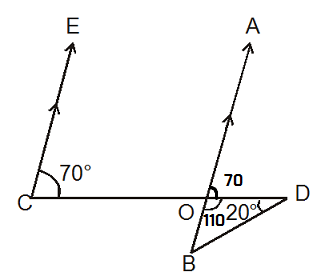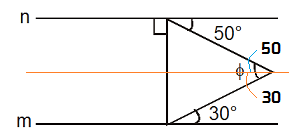# Geometry - Lines

Geometry is basically the study of different properties of point, line and plane.

Angles:
Right angle: An angle of 90° is called a right angle.
Acute angle: An angle of less than 90° is called an acute angle.
Obtuse angle: An angle of greater than 90° is called an obtuse angle.
Reflex angle: An angle of greater than 180° is called reflex angle.
Complementary angles: If the sum of the two angles is 90°, then they are called complementary angles.
Supplementary angles:  If the sum of the two angles is 180°, then they are called supplementary angles.

Angle Relations:

Vertically opposite angles: If two lines intersect each other, then the vertically opposite angles are equal.
$\angle$a = $\angle$c and $\angle$b = $\angle$d
a, c and b, d are vertically opposite angles.Angles in parallel lines: If a transversal intersects two parallel lines:a. each pair of consecutive interior angles are supplementary.
$\angle$4 + $\angle$5 = 180°
$\angle$3 + $\angle$6 = 180°

b. each pair of alternate interior angles are equal.
$\angle$5 =$\angle$3, $\angle$6 = $\angle$4
$\angle$5 = $\angle$3 =$\angle$1 =$\angle$7
$\angle$6 = $\angle$4 = $\angle$2 = $\angle$8

Collinear: Three or more than three points are said to be collinear, if there is a line which contains them
all.
Concurrent: Three or more than three lines are said to be concurrent, if there is a point which lies on them
all.

Proportionality Theorem:In the above diagram, $\displaystyle\frac{{AB}}{{BC}} = \frac{{DE}}{{EF}}$ or $\displaystyle\frac{{AC}}{{BC}} = \frac{{DF}}{{EF}}$

Solved Examples:

1. In the adjoining figure, find $\angle$ C. If AB // CDSol:   $\angle$ E = 180 - 120 = 60$\angle$ E + $\angle$ X + $\angle$ F = 180 $\Rightarrow$ 60 + 50 + F = 180 $\Rightarrow$ F = 70
Now $\angle$ G = 180 - F = 110.
As AB // CD, $\angle$G = $\angle$C
So $\angle$C = 110

2. Find the value of x in following figure.Sol: As C // E, $\angle$B = $\angle$YAlso $\angle$Y + $\angle$Z = 180 $\Rightarrow$  $\angle$Z = 180 - 130 = 50
Now in $\Delta$ AGF, $\angle$A + $\angle$Z + $\angle$X = 180  $\Rightarrow$ $\angle$X = 180 - 20 - 50 = 110

3. Find $\angle$OBD in the given figure. It is given that EC || AO.Sol:   From the diagram, in $\Delta$OBD, 110 + 20 + $\angle$B = 180 $\Rightarrow$ $\angle$B = 504. If the two lines n and m are parallel, then find the value of $\phi$ in the given figure.Sol:  Draw a parellel to n. Now from the diagram $\phi$ = 50 + 30 = 80 (Alternate interior angles are equal)Alternate method:  you can also solve this problem by calculating interior angles of the triangle.